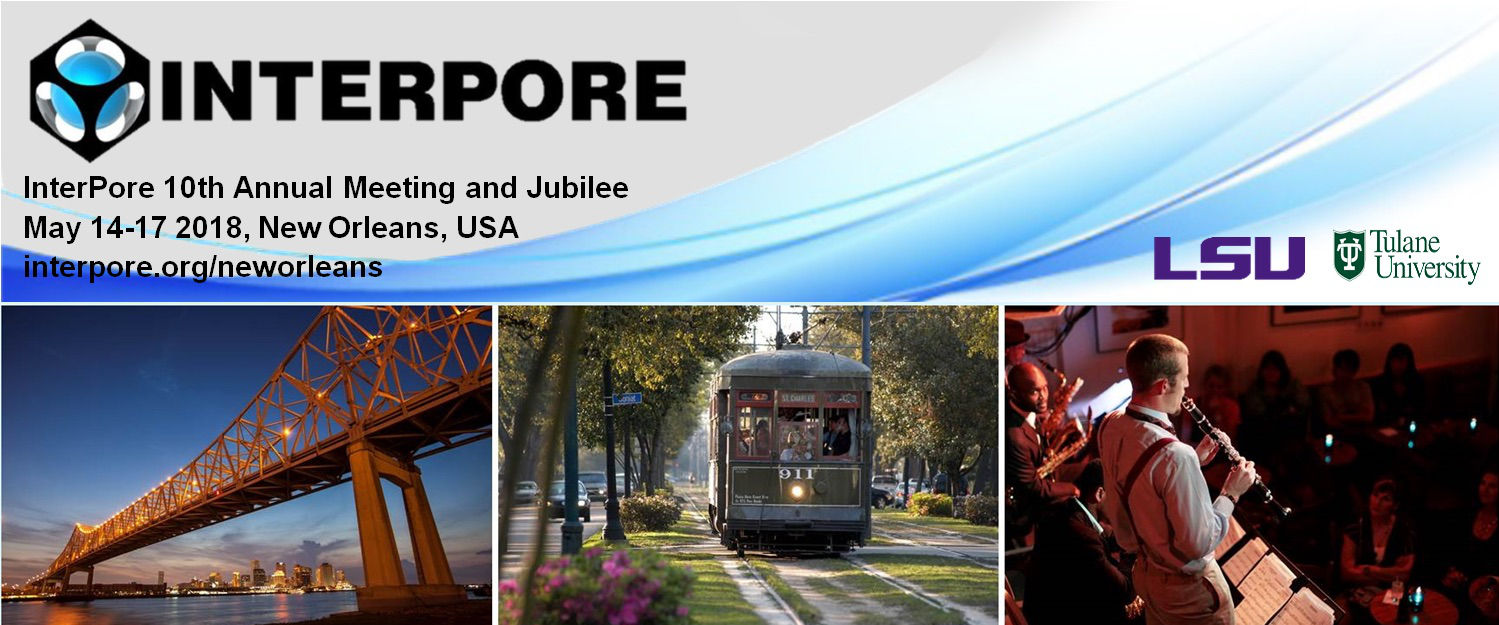#InterPore2018 New Orleans

14-17 May 2018
New Orleans
US/Central timezone

## Numerical methods for non-equilibrium porous media flow models

16 May 2018, 15:58
15m
New Orleans

#### New Orleans

Oral 20 Minutes MS 2.12: High order schemes for simulation of flow and transport in porous media

### Speaker

Iuliu Sorin Pop (Hasselt University)

### Description

Standard models for flows in porous media assume that quantities like saturation, phase pressure differences, or relative permeability are related by monotone, algebraic relationships. These relationships are determined experimentally, assuming that the involved quantities have reached a local equilibrium. Under such assumptions, the solutions of the resulting mathematical models have properties like stability in various norms, and satisfy the maximum principle. In particular, standard models rule out effects like saturation overshoot or the formation of finger profiles, which have been evidenced experimentally. This contradiction is the main motivation when considering non-equilibrium models, where dynamic effects are included in the above mentioned relationships. The resulting models are nonlinear evolution systems of (pseudo-)parabolic and possibly degenerate equations, and may involve differential inclusions. In this contribution we discuss different numerical schemes (multipoint flux approximation, discontinuous Galerkin) for approximating the solution of non-equilibrium models. In particular, we present the rigorous convergence analysis of the numerical solution to the weak solution of the model. Also, we analyze different linear iterative methods for approximating the numerical solution to the nonlinear, fully discrete problems encountered at each time step.

### References

X. Cao, S.F. Nemadjieu, I.S. Pop, A multipoint flux approximation finite volume scheme for two phase porous media flow with dynamic capillarity, IMA J. Numer. Anal. (accepted).

S. Karpinski, I.S. Pop, F.A. Radu, Analysis of a linearization scheme for an interior penalty discontinuous Galerkin method for two phase flow in porous media with dynamic capillarity effects, Internat. J. Numer. Methods Engrg. Vol. 112 (2017), pp. 553–577.

S. Karpinski, I.S. Pop, Analysis of an interior penalty discontinuous Galerkin scheme for two phase flow in porous media with dynamic capillarity effects, Numer. Math. Vol. 136 (2017), pp. 249-286.

F.A. Radu, K. Kumar, J.M. Nordbotten, I.S. Pop, A robust, mass conservative scheme for two-phase flow in porous media including Hoelder continuous nonlinearities, IMA J. Numer. Anal. (accepted).